# Microwave Phase Shifters, IQ Vector Modulators and Frequency Translators: Application Notes

Request More Product Info | Phase Shifters Selection Guide | IQ Vector Modulators Selection Guide | Frequency Translator Selection Guide

## Introduction to Microwave Phase Shifters

Kratos General Microwave Corporation has been a leader in the field of microwave PIN diode control components for more than 35 years. The design and manufacture of high performance, broadband phase shifters, frequency translators and I-Q Modulators have made Kratos General Microwave the undisputed leader for these devices.

Today’s more demanding systems require the ability to control the phase and amplitude of RF/microwave signals with a repeatable, high degree of accuracy. Kratos General Microwave intends this section to not only inform you of our most popular products but also to provide insight into theory of operation, calibration and practical applications where they can be utilized.

Kratos General Microwave offers a complete line of broadband phase shifters and I-Q modulators which span the frequency range from 0.05 to 40.0 GHz. These devices are available in several different topologies that allow the designer to choose among various performance characteristics that best suit his system needs. This section describes only our standard line of broadband phase shifter and I-Q modulator models. In addition to these, there are numerous special designs, employing a variety of phase shifter circuits, which Kratos General Microwave has utilized in custom applications.

###### PHASE SHIFTER FUNDAMENTALS
A variable phase shifter can be characterized as a linear two port device which alters the phase of its output signal in response to an external electrical command. (Mechanical phase shifters are not considered here.) Expressing this mathematically, with an input signal sin (ωt), the output will be A(n)*sin[(ωt+Θ(n)], where n is the programmed phase and A(n) is the insertion loss. The difference between the input phase and the output phase is the sum of the phase shift due to the propagation through the phase shifter plus the programmed phase shift.
The relative simplicity of the idea that any reactance placed in series or shunt with a transmission line will produce a phase shift has given rise to many different circuits over the years for use as phase shifters at microwave frequencies.

Usually, for high speed applications, the controlling elements have been semiconductor devices such as PIN, Schottky and varactor diodes, whereas for high power requirements, when slower switching speed can be tolerated, ferrites are frequently employed. The final choice of a phase shifter network and control element will depend on the required bandwidth, insertion loss, switching speed, power handling, accuracy and resolution. In addition, a choice between analog and digital control must also be made. Analog phase shifters are devices whose phase shift changes continuously as the control input is varied and therefore offer almost unlimited resolution with monotonic performance. The most commonly used semiconductor control devices used in analog microwave phase shifters are varactor diodes, which act as current controlled variable resistors. Schottky diodes and ferrite devices are also used as variable elements in analog phase shifters but the former suffer from limited power handling capability and matching difficulty in broadband networks whereas the latter are generally larger, require more bias power, and are relatively slow compared to semiconductor designs. Among the more useful topologies for analog phase shifters are the loaded line design using lumped or distributed elements and the reflective design employing quadrature hybrids. One of the variants of the reflective phase shifter is the vector modulator, which in the particular embodiment used by Kratos General Microwave shows excellent performance over 3:1 bandwidths. This capability is especially useful in the design of frequency translators(1) and high resolution phase shifters for EW systems as well as in broadband simulators as I-Q modulators, where separate control of the quadrature components of the signal allow for independent adjustment of both phase and amplitude. Analog phase shifters are readily convertible to digital control by the addition of suitable D/A converters and appropriate linearizing circuits. (1)Phase shifters can be used to translate the frequency of an RF carrier by subjecting it to a linear time varying phase shift.
###### (1)Phase shifters can be used to translate the frequency of an RF carrier by subjecting it to a linear time varying phase shift.WHAT IS AN IQ VECTOR MODULATOR?
An IQ Vector Modulator is an RF or microwave circuit which has the ability to control both the amplitude and phase of the transmitted signal simultaneously. Any sinusoidal signal can be expressed as a vector having the properties of both amplitude and phase with respect to a reference signal. If a signal is thought of as a vector in a polar coordinate system with coordinates of amplitude and phase, it can also be defined in a rectangular coordinate system with coordinates of “I” and “Q”. The term “IQ” does not represent anything about the intelligence of the design engineer, but rather that the user can control both the “In-Phase” and “Quadrature-Phase” components of the output signal.
###### WHAT IS A TYPICAL IQ MODULATOR CIRCUIT?
The circuit typically includes an input power divider which splits the incident signal into two paths, an amplitude and/or phase control element in each path, and an output signal summing circuit. In the simplest embodiment, the input signal is divided into two equal signals with a 90° phase difference; controlled by a phase invariant bi-phase attenuator in each path; and combined by an in phase power combiner as shown in figure 1.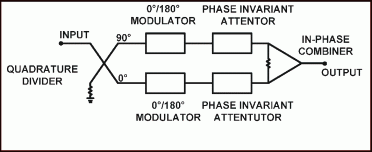FIGURE 1
I-Q Vector Modulator Block diagram
###### WHAT ACTIVE CONTROL COMPONENTS ARE USED IN IQ MODULATORS?
The control components in an IQ vector modulator are circuits that employ PIN diode, Schottky diode or FET devices. The simplest circuit uses a PIN diode attenuator in series with a PIN-diode bi-phase modulator, or a combination of the two devices in a single bi-phase attenuator. This device has the property of providing a continuous function which first attenuates the input signal with no phase shift, then shifts phase 180° at maximum attenuation, and then decreases attenuation while holding a constant 180° phase shift. Balanced or double balanced Schottky diode or FET mixers exhibit a similar function, but are limited in dynamic range of attenuation. PIN diode devices usually exhibit higher power handling, lower insertion loss and higher intercept points than Schottky diode or FET based devices. Schottky diode or FET devices are preferred for modulation rates higher than a few megahertz.
###### WHAT ARE SOME OF THE USES OF IQ VECTOR MODULATORS?
• Amplitude and Phase control for RF simulator systems
• Cancellation of unwanted jamming signals
• Cancellation of crosstalk between co-located communication systems
• Cross-Polarization Cancellation
• Doppler Simulation
• Nulling of antenna reflections in monostatic radar systems
• Complex weights for Phased Array Antennas
• Linear Filter Equalizer
###### HOW ARE IQ VECTOR MODULATORS CALIBRATED?
Calibration of the IQ vector modulator for controlled amplitude and phase response is often performed by generating a “look-up” table using a vector network analyzer. To obtain the highest degree of accuracy, the calibration should be performed in-situ. A discussion of calibration techniques is provided later on. When IQ vector modulators are used in a nulling system an algorithm can readily be developed to adjust the values of I and Q in a closed loop fashion to achieve the desired system performance.
###### CAN THE I-Q VECTOR MODULATOR BE CUSTOMIZED FOR SPECIAL APPLICATIONS?
Kratos General Microwave has customized many variations of the IQ vector modulator for numerous applications ranging from low cost designs to nuclear hardened radar systems. Our sales and engineering staff are available to help you maximize your system performance by incorporating IQ vector modulators to meet challenging system requirements.
##### DEFINITION OF PARAMETERS
Phase Shift: The difference in phase angle of the existing RF signal at a given frequency and phase shift setting referenced to the exiting signal at the same frequency with the phase shifter set to zero degree phase shift. Accuracy: The maximum deviation in phase shift from the programmed phase shift over the operating frequency range when measured at room temperature. Temperature Coefficient: The average rate of change in phase shift, as referenced to the zero degree phase state, over the full operating temperature range of the unit. Expressed in degrees phase shift/degrees C. PM/AM: The maximum peak-to-peak change in insertion loss of the phase shifter at any phase state over the full 360° phase range. Switching Speed: The time interval from the 50% point of the TTL control signal to within 10° of final phase shift. This applies to a change in either direction between any two phase states which differ by more than 22.5°. Carrier Suppression: When the phase shifter is operated as a frequency translator, the minimum ratio of carrier output power to the translated carrier output power. Sideband Suppression:

When the phase shifter is operated as a frequency translator, the minimum ratio of any sideband output power to the translated carrier output power.

Translation Rate:

When the phase shifter is used as a frequency translator, the translation rate is determined by dividing the clock rate by the number of steps. Number of steps is equal to 2n where n equals number of bits.

Frequency Range

Phase Shifters/Frequency Translators are specified to operate over a very broad frequency range. In order to optimize performance, the total frequency range has been broken into a Main Band and a Stretch Band. The Stretch Band frequency range segments are typically above and below the Main Band frequency range.

Main Band:

Phase Shifters/Frequency Translators are factory set to meet the more accurate performance shown in the Main Band specifications. If operated as factory set, but outside the Main Band frequency limits, the performance will me more typical of that shown for the Stretch Band specifications.

Stretch Band:

Phase Shifters/Frequency Translators performance, outside the Main Band frequency limits, can be improved by utilizing the Frequency Correction Bit. This is done by the application of a TTL Low (0) at Pin 3 of the Power/Control Connector J3. Once done, the performance in the Stretch Band frequency segments will be enhanced to meet the specifications shown for the Main Band of operation.

##### TYPICAL PERFORMANCE CHARACTERISTICS
###### HARMONICS AND INTERMODULATION PRODUCTS

All PIN diode control devices will generate harmonics and intermodulation products to some degree since PIN diodes are non-linear devices. When compared to digital switched-bit designs, analog PIN diode phase shifters are more prone to generate spurious signals since the diodes function as current-variable resistors and are typically operated at resistance levels where significant RF power is absorbed by the diode. The levels of harmonic and intermodulation products generated by a phase shifter or I-Q modulator are greatly dependent upon its design, the operating frequency, attenuation setting and input power level. Typical 2nd and 3rd order intercept performance for a moderately fast phase shifter, i.e. 500 nsec switching speed follows:

 TYPICAL INTERCEPT POINTS Frequency 2nd Order Intercept 3rd Order Intercept 2.0 GHz 8.0 Ghz +35 dBm +40 dBm +30 dBm +35 dBm
###### PHASE NOISE

The phase shifters and I-Q modulators offered by Kratos General Microwave minimize the contribution of phase noise to system performance. This is accomplished by utilizing PIN diodes which are less sensitive to high frequency noise than Schottky diodes, limiting the noise bandwidth in driver control elements and the use of low noise buffer amplifiers to drive the PIN diodes.

###### WHAT IS FREQUENCY TRANSLATION?

Translation is shifting the frequency of a signal by a user controlled delta. This frequency delta, also known as Translation Rate, is usually notated by “Fm”.

When the user wants to translate the signal by 1 Hz he needs to apply a ramp (counter) that sweeps the phase control of the phase shifter (translator) starting from zero phase shift and ending at 360° in a cyclic manner.

Each cycle should take exactly 1 second in order to achieve a shift of 1 Hz. Using 10 bit counter, the clock of the counter would be 1/1024 Hz. Using only 5 bit counter, the clock of the counter would be 1/32 Hz. So using less bits will enable lower clock rates. However, too low number of bits will cause poor sidebands and carrier suppression.

Let us assume that we have a pure sine-wave signal as a carrier at Fc that appears at the output of the phase shifter with a nominal amplitude of 0 dBm. Now, when introducing an Fm [Hz] translation (covering the 360º once every 1/Fm [seconds]) using the 5 Most Significant Bits.
With a perfect phase shifter we expect that the spectrum will look like this: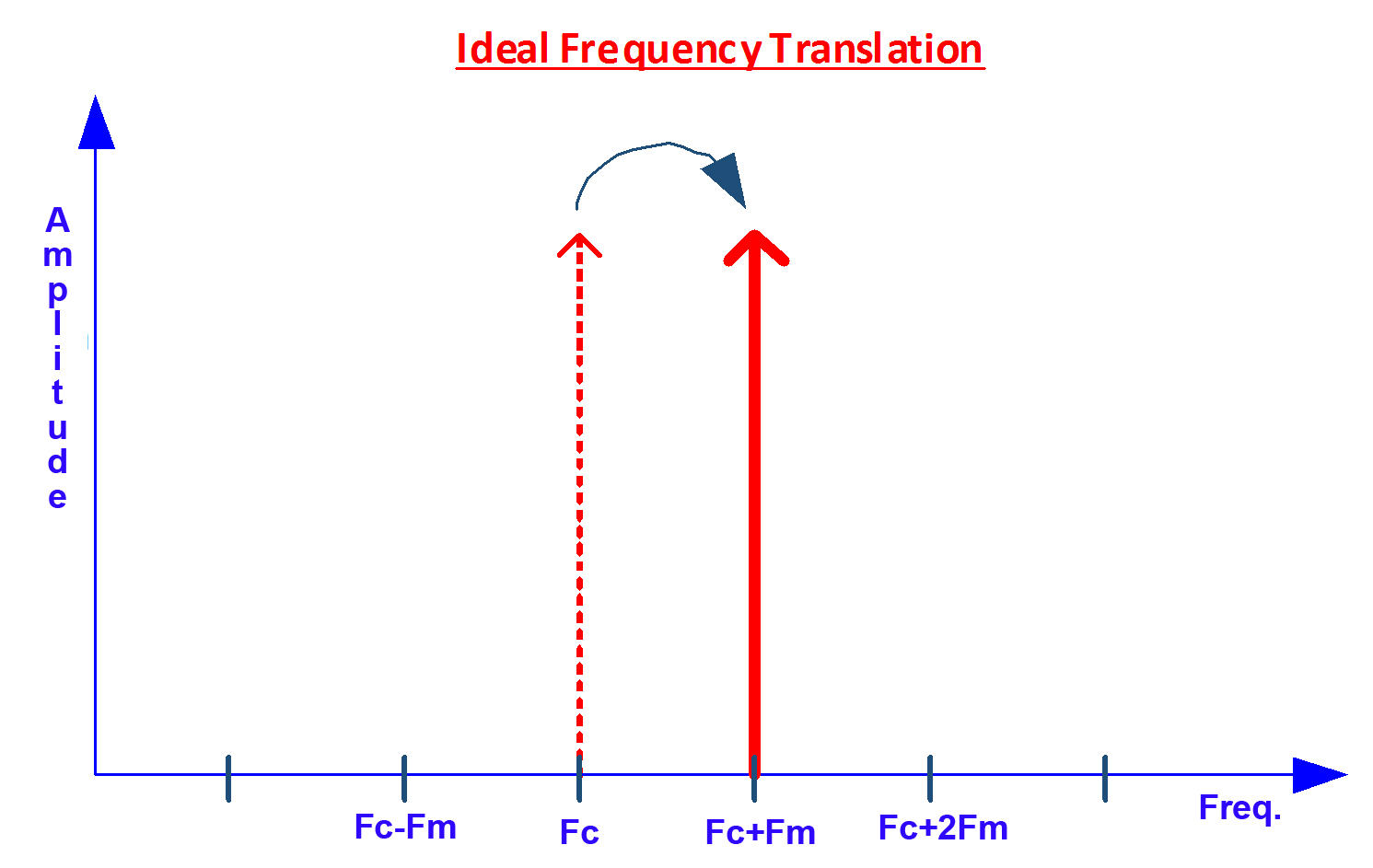However, with a practical phase shifter the spectrum will look like this: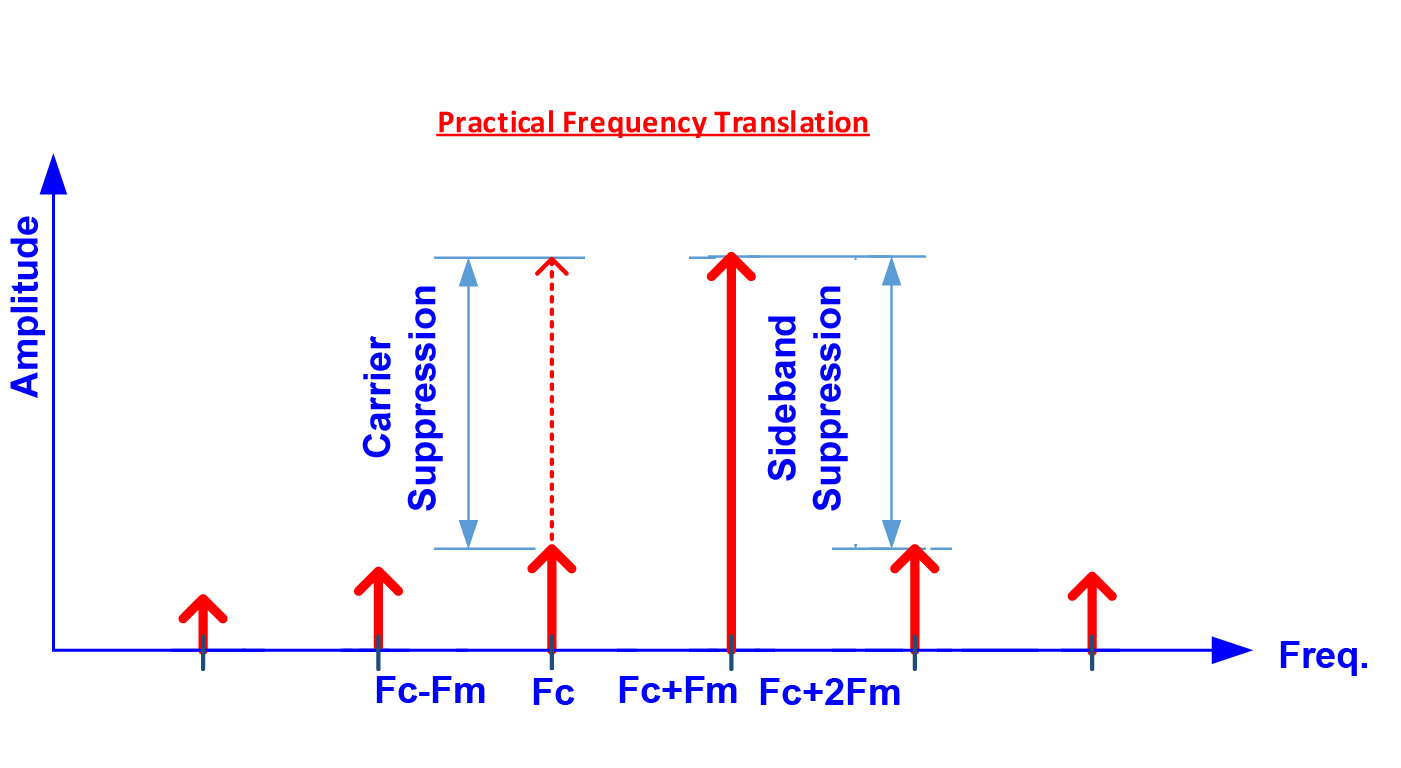Where:

Carrier Suppression: The amplitude difference between the translated signal and the original carrier. Sideband Suppression: The amplitude difference between the translated signal and the strongest sideband (could be at Fc-n*Fm or Fc+n*Fm, but usually is the Fc+2*Fm product).

IL Variation: Reduction of the Translated Carrier amplitude relative to an amplitude at low translation rate (50 kHz).”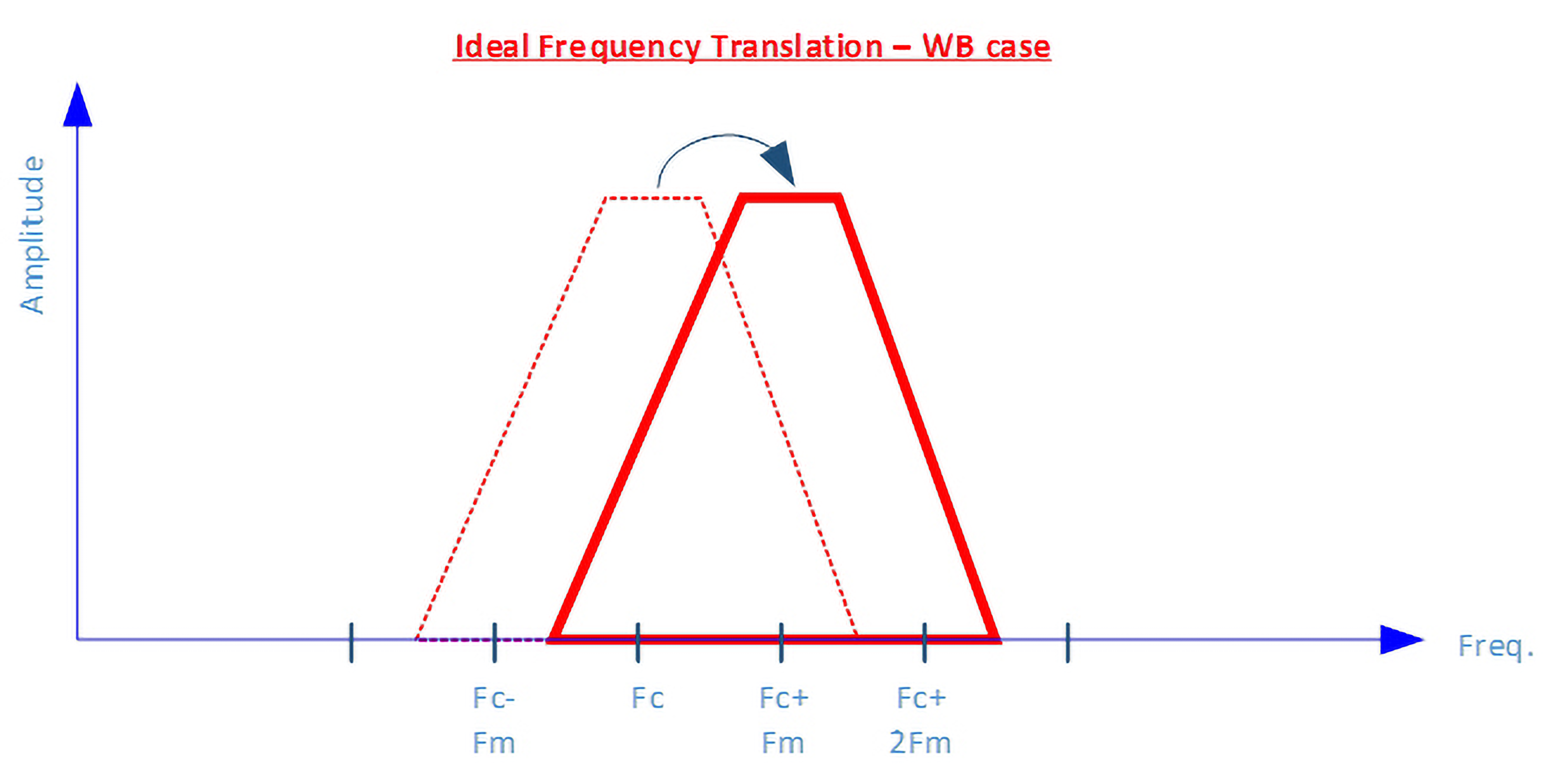###### THEORY OF OPERATION & PRACTICAL APPLICATIONS

I-Q VECTOR MODULATOR —

THE IDEAL CONTROL COMPONENT!
Microwave control components are used to vary signal amplitude and phase. Typically, they consist of two- port devices including amplifiers, attenuators, phase shifters, and switches. The I-Q vector modulator is a unique combination of active and passive devices that is, in theory, ideally suited for the simultaneous control of amplitude and phase.

###### THEORY OF OPERATIONFIGURE 1
I-Q Vector Modulator Block diagram

The block diagram of the I-Q vector modulator is shown in Figure 1. An RF signal incident on a 3 dB quadrature hybrid is divided into two equal outputs, with a 90 degree phase difference between them. The in-phase or 0 degree channel is designated the I channel and the quadrature or 90 degree channel is designated the Q channel. Each signal passes through a biphase modulator which selects the 0 or 180 degree state for both the I and the Q paths. This defines the quadrant in which the resultant output signal resides (Figure 2). The attenuator in each path then varies the magnitude of each of the signals, which are combined in phase to yield the resultant vector. This vector will lie anywhere within the bounded area shown in Figure 2. Thus, any signal applied to the l-Q vector modulator can be shifted in phase and adjusted in amplitude by assuming the desired attenuation level = x dB and the desired phase shift = Θ degrees. The normalized output voltage magnitude is then given by:

R = 10–(x/20) The attenuation values of the I and Q attenuators are then given by:

I attenuator (dB) = 20 log (R cos Θ)
Q attenuator (dB) = 20 log (R sin Θ)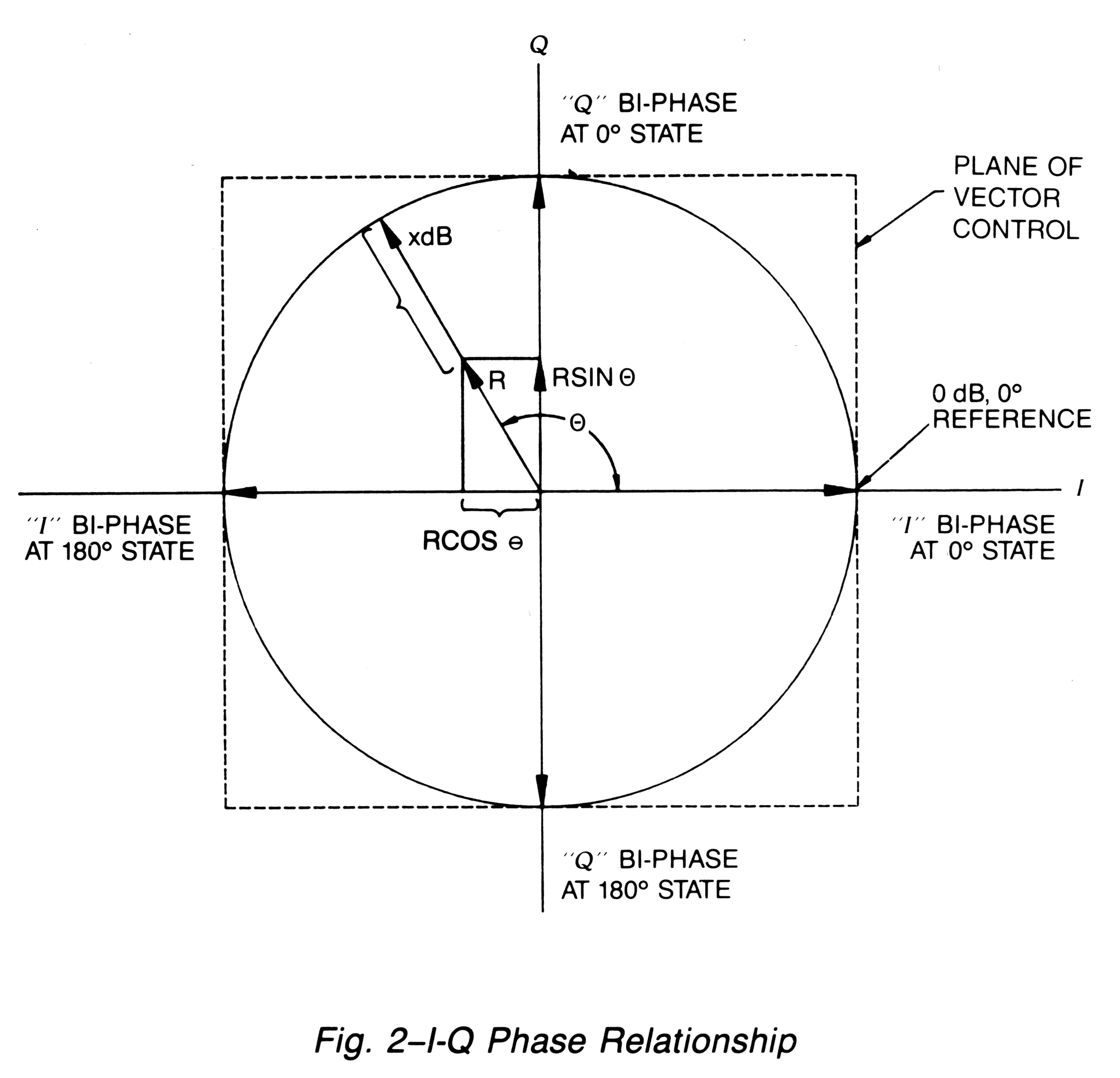To achieve the desired phase shift, bi-phase modulator states must also be selected as shown in Table 1. In this way, the phase and amplitude of the output signal can be varied simultaneously in a controlled fashion.

 TABLE 1 Bi-phase Modulator States Desired Phase Shift I Q 0° 0° 0°-90° 180° 0° 0°-180° 180° 180° 180°-270° 0° 180° 270° -360°

The theoretical model presupposes perfect amplitude and phase balance in the two signal paths, and ideal quadrature coupling in the 3 dB hybrid. To the extent that the conditions are not met in practice, the performance of the I-Q vector modulator will be limited.

PHASE BALANCE

The key element in determining the useful frequency range of the I-Q vector modulator is the 3 dB quadrature hybrid. Its most important characteristic is very low quadrature phase error (such as small deviation from 90 degree phase shift between outputs). To achieve this over a broad frequency range, we employ the Hopfer quadrature hybrid(2), which exhibits extremely wideband quadrature-phase properties (typically greater than 3 to 1 bandwidth with ±2 degree phase balance). In addition to using an in-phase Wilkinson combiner (which, with proper design, exhibits excellent phase balance) the transmission-line length for the I and Q paths must also be carefully phase-matched.

(2) S. Hoofer, “A Hybrid Coupler for Microstrip Configuration,” IEEE MTT-S International Microwave Symposium Digest, 1979.

AMPLITUDE BALANCE

The amplitude balance of the I and Q paths is a second source of performance limitation. Unequal power levels in these paths also produce errors in both the amplitude and phase of the transmitted signal. To minimize this source of error, the quadrature hybrid coupling must be adjusted to provide minimum deviation from the nominal 3 dB across the frequency band. For an ideal hybrid, the amplitude unbalance will be ±0.31 dB over an octave band. The effect of amplitude and balance error on phase is shown in Figure 3.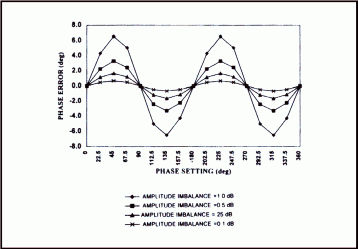FIGURE 3 Phase Error Due to Amplitude Imbalance Non-IDEAL BI-PHASE MODULATOR AND ATTENUATOR

Errors in amplitude and phase will occur if the biphase modulator deviates from the ideal, eg: changes state from 0 to 180 degrees with constant amplitude or if the attenuator has an associated phase shift as attenuation is varied. Not only do these components in practice exhibit such deviations, but their interacting reflections may increase the resultant errors significantly. The arrangement in Figure 4 minimizes the errors. As indicated, the tandem combination of a biphase modulator and attenuator in each path is replaced by a doubly-balanced biphase modulator. The doubly-balanced biphase modulator developed by Kratos General Microwave(3) has the ability to attenuate a signal by more than 20 dB with constant phase, then change the phase 180 degrees and return to the low-loss state. At insertion loss, it exhibits a maximum phase error of less than ±6 degrees and an amplitude balance of ±0.5 dB over a 3 to 1 bandwidth.

(3) Z. Adler and B. Smilowitz, “Octave-Band High-Precision Balanced Modulator,” IEEE MTT-S International Microwave Symposium Digest, 1984.

###### PRACTICAL APPLICATIONS
PHASE SHIFTERS

If the doubly-balanced biphase-modulator conditions are adjusted so that the magnitude of the resultant vector remains fixed, the I-Q vector modulator can behave as a constant-amplitude phase shifter. The relationships between the desired phase shift and the I and Q attenuation levels are given by:
I = cos Θ
Q = sin Θ
where I and Q are normalized voltages.

3) Z. Adler and B. Smilowitz, “Octave-Band High-Precision Balanced Modulator,” IEEE MTT-S International Microwave Symposium Digest, 1984. Theory of Operation & Practical Applications

The relationship between the I and Q drive circuitry can be generated in either analog or digital fashion. The analog circuit employs a broadband quadrature hybrid to generate the drive signals. In the digital drive circuit, PROMS are used to provide the required relationships between I and Q. See the Selection Guide on page 61 for the Kratos General Microwave phase shifter model numbers.

###### FREQUENCY TRANSLATORS

A signal-processing technique using a linear time varying phase shifter is one method of frequency translation. One principal use is in velocity deception for ECM systems by providing false Doppler radar returns. In a true Doppler radar situation, the reflected signal is translated in frequency in an amount proportional to the radial velocity of the target. As a rule, there are no harmonics or spurious signals accompanying the reflection. However, if the target is using velocity deception techniques, spurious signals may be present in the radar return because of the non ideal performance of the frequency translator. The presence of these spurious signals will reveal that the Doppler radar is being jammed. Therefore, it is critical for optimum ECM system performance that the frequency translator suppress the carrier, harmonics and all unwanted sidebands to the greatest extent possible. For the linear phase shifter, the principal factors that contribute to imperfect carrier suppression and sideband generation are:

2π error

This is the deviation from 360 degrees when maximum phase shift is programmed.

PM/AM error

The amplitude change (AM) is a function of the phase change (PM).

Phase nonlinearity

It is the deviation from linear phase shift vs. time.

Quantization error

This term is usually negligible for phase resolution greater than 6 bits. It arises in a digital phase shifter, which only approximates linear phase shift with discrete phase steps.

Flyback time

This arises from the finite time required by the phase shifter to return from 360 to 0 degrees.

In the I-Q modulator, since the network operates as a constant-velocity rotating vector, the 0 and 360 degree phase states are exactly the same, and the 2π error and flyback error are eliminated. In addition, the Kratos General Microwave Series 77 provides 10 bits of digital phase control (sufficient to eliminate the quantization error), while phase linearity is optimized by the use of PROM correction in the drive circuitry. Finally, the PM/AM error is minimized by using matched doubly-balanced biphase modulators, thereby reducing this error essentially to the difference in amplitude of the 3 dB quadrature hybrid output ports. This amplitude imbalance varies with frequency and generates a unique spurious sideband during frequency translation. An additional PROM correction using RF operating frequency information can be employed to reduce this spurious sideband for customer requirementsץ

The specifications of the Kratos General Microwave Series 77 Digitally Controlled and Series 78 Voltage Controlled Frequency Translators include 25 dB carrier suppression and 20 dB sideband suppression over a three-to-one frequency range. Typical performance data for carrier and sideband suppression, of the 6 to 18 GHz Model 7728A, are shown in Figure 5. Carrier and sideband suppression of greater than 34 dB for a frequency translator covering a 15-percent bandwidth at X band over the operating temperature range of –54°C to +100°C have been achieved in production quantities.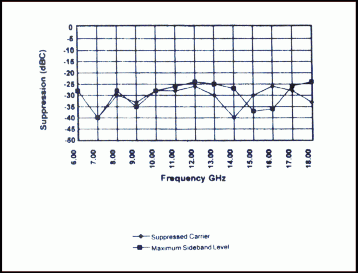Figure 5 -Typical Carrier and Sideband Suppression Kratos General Microwave Model 7728A Frequency Translator

COMPLEX I-Q VECTOR MODULATORS

System requirements often call for a tandem connection of phase shifters and attenuators to provide independent control of magnitude and phase of an RF signal. If tight tolerances are required for the amplitude and phase accuracy, a look-up table is usually incorporated in the system software to calibrate the phase shift and attenuation across the frequency range. This is a tedious job that entails the generation of an extensive amount of error correction data, obtained by alternately varying the phase shifter and attenuator over the dynamic range for each narrow frequency band where optimization is required. The inclusion of an I-Q vector modulator in the system in place of a discrete phase shifter and attenuator offers several distinct advantages. A single RF component replaces two separate units, thus reducing cost and eliminating interacting VSWR. The relationship between the I and Q inputs and the desired amplitude and phase permits a tremendous reduction in the amount of data required for a look-up table. This is because the I and Q inputs are independent variables for the I-Q vector modulator, whereas the tandem connection of attenuator and phase shifter exhibit large AM to PM and PM to AM pushing, creating dependency between the amplitude and phase inputs. Depending on the frequency range and accuracy specifications, the RF circuitry of the I-Q vector modulator can be optimized to eliminate the need for a look-up table entirely.

The I-Q Vector Modulator is ideally suited for use in EW Simulators, Adaptive Equalizers or Automatic Test/ Calibration Systems where extremely high accuracy and repeatability are essential.

##### Amplitude and Phase Calibration

Kratos General Microwave I-Q Vector modulators can be calibrated to provide precision control on both amplitude and phase over their full rated dynamic range. The calibration is performed using a vector network analyzer and a customer generated test program to achieve the utmost in accuracy. The most frequently used algorithm to accomplish this calibration is described herein. This algorithm involves defining a unity circle and then employing an iterative technique to locate precise calibration values. Many factors contribute to the overall accuracy that is achievable using any calibration routine for the I-Q vector modulator. It is important that the user fully understand the limitations of measurements in calibrating these units at microwave frequencies. For example, it is imperative that the desired calibration accuracy not exceed the accuracy and repeatability of the microwave test equipment. Another factor which must be included in the overall calibration accuracy is the effects of temperature on the I-Q modulator and the test equipment. Given that the user has a thorough understanding of vector network analyzer measurements, the following will be useful for generating a calibration program for a digitally controlled I-Q vector modulator. (Note that an analog controlled unit can be calibrated in the same fashion using the relationship that 000 hex equals zero volts and FFF hex equals ten volts on the I and Q controls.)

1.0 The calibration routine is performed at discrete frequencies in the band of interest. The calibration will be valid over an interval of frequencies centered at the calibration frequency and will be limited by the amplitude and phase errors that occur as frequency is varied. The highest calibration accuracy will occur with minimum frequency interval size. However this may require an excessive amount of calibration time and data storage. It is recommended that a calibration interval of 100 to 200 MHz be used in the center of the frequency range of the vector modulator and 25 to 50 MHz be used at the band edges. The optimum calibration interval for any user must be determined empirically by insuring that the maximum phase and amplitude error over the frequency calibration interval is within the desired limits.

2.0 Once the calibration interval and the calibration frequency have been chosen, the next step is to define the I and Q axes and the magnitude of the unit circle. For this example, the I axis is defined to be the horizontal axis on the I-Q plane with control word 000 (hex) being equivalent to a vector of approximate magnitude 1.0 at an angle of zero degrees. In the same fashion the Q axis is defined to be the vertical axis on the I-Q plane with control word 000 (hex) equivalent to a vector of approximately magnitude 1.0 at an angle of 90 degrees. Note that for both I and Q, the magnitude zero vector is approximately 7FF (hex) and the magnitude –1.0 vector occurs as FFF (hex). Following this procedure the definition of the I-Q plane is arrived at per the table below:

 TABLE 2 I CONTROL (hex) Q CONTROL (hex) APPROX. VECTOR 000 7FF 1.0 ANG 0° FFF 7FF 1.0 ANG 180° 7FF 000 1.0 ANG 90° 7FF FFF 1.0 ANG 270°

3.0
The magnitude of the unit circle is determined by finding the maximum insertion loss at the calibration frequency in each of the four states in table 2 above. Since by nature the I-Q plane is a square and not a circle (see figure 6), the maximum insertion loss will occur at one of these four states. Once the maximum insertion loss is determined, the I or Q values of the other three states in table 2 are adjusted to meet the same maximum insertion loss level. Note that only either I or Q should be adjusted to increase insertion loss at any state, not both. The I or Q value that is initially set to 7FF (which is approximately the center of the IQ plane) is not varied during this part of the calibration since the amplitude of the unit circle is not affected by small changes in the control input.

4.0 Having thus defined the unit circle, the next step is to scale the I and Q axes to allow for computation of I and Q values given the desired amplitude and phase. If the I and Q axes were perfectly linear and each consisted of 4096 equal increments (for a 12 bit control), it would be possible to achieve the desired amplitude and phase shift using only the sine and cosine relationships given in figure 6. In order to approach the ideal case, the I and Q values for each of the four states given in table 2 must be scaled if they differ from 000 or FFF (note that the control input at 7FF is not varied in this step). The scaling entails taking the difference between 2048 digital counts (equal to one half of the 12 bit control) and the number of counts required to equalize the insertion loss of each of the four states required for the unit circle derived from step 3.0. For example, assume that the I value at zero degrees (I=000, Q=7FF), is the maximum insertion loss of the four states and that in order to achieve the same level of insertion loss at 180° (nominal value I=FFF, Q=7FF), I must be lowered by 127 counts such that the new value for 180° on the unity circle is I=F80, Q=7FF. In this case the I axis for I<0 (in the second and third quadrants) is limited to 1921 counts instead of 2048. Thus, when the algorithm is determining the equivalent I value for a desired amplitude and phase occurring in the first or fourth quadrants, the calculated value for I=R*cos Θ is multiplied by 2048 and the result subtracted from 2048 (1=7FF, the origin). When, in the same example, this calculation is done for a vector that occurs in the second or third quadrants, the calculated value for I= R*cos Θ will be multiplied by 1921 and the result added to 2048 (I=7FF) to find the desired I value (reference the I scale at the bottom of figure 6). The scale value will be called SCALE in calculations given in step 5.2. While this scaling is not precise, it is sufficient to enable the algorithm to establish the boundary of the I-Q plane such that any desired amplitude and phase calibration point can be achieved with a minimum of iterations.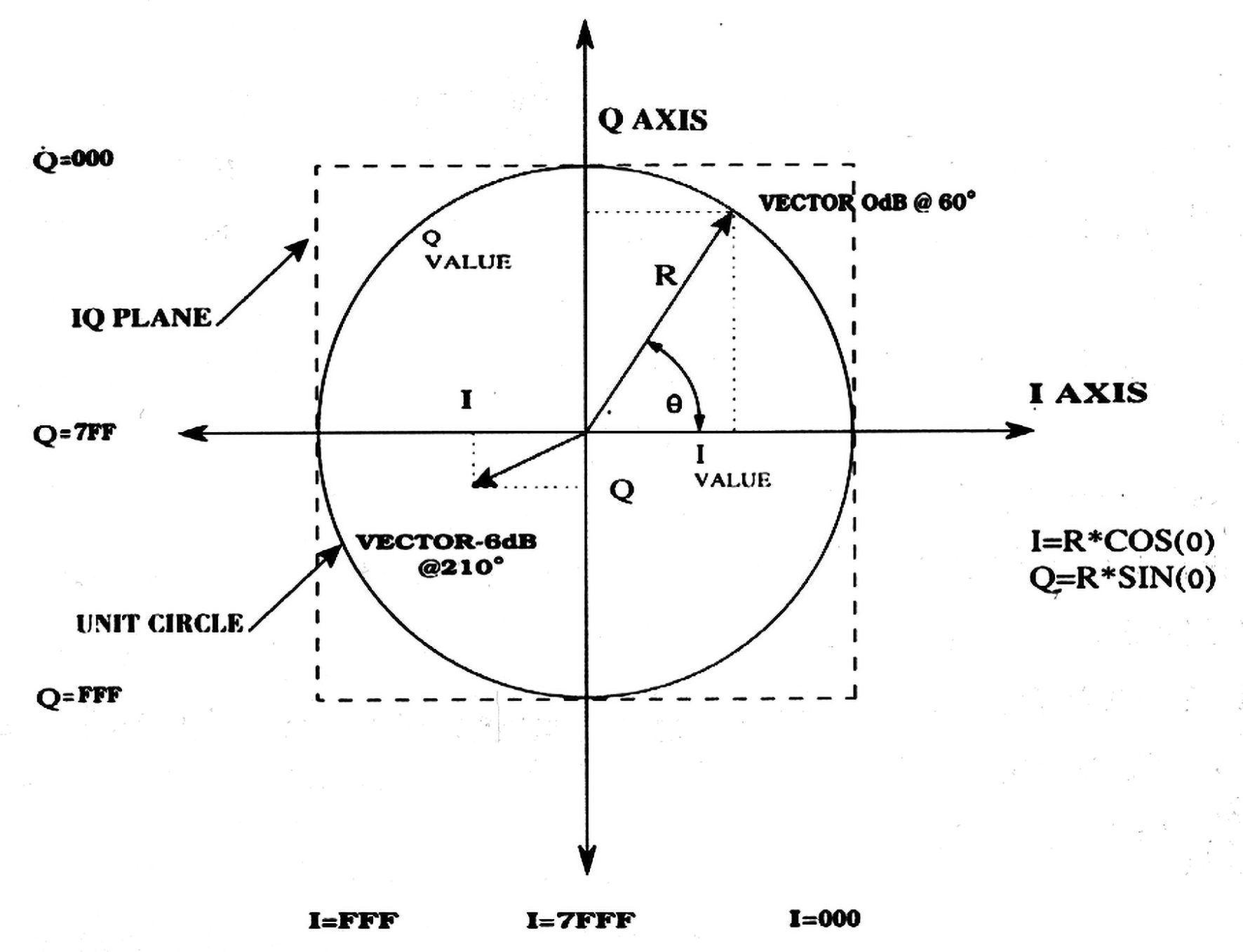Figure 6
I-Q Vector Model

5.0 Once the scaling of the axes has been accomplished, the zero degree point on the unity circle is stored and normalized on the vector analyzer. The control word for this point will be approximately I=000, Q=7FF and all succeeding phase and amplitude values will be referenced to this point. Note that the I control word will differ from 000 if it is not the maximum insertion loss state of the four states listed in table 2. The Q control word will be equal to 7FF. An algorithm to find any desired amplitude and phase with respect to the normalized unit circle zero degree point can be constructed from the following procedure:

5.1 Convert the desired amplitude to a ratio such that the desired amplitude and phase can be expressed as a magnitude (R) and phase (Θ). This is the desired phase and amplitude change with respect to the normalized point obtained in step 5.0.

5.2 Solve for the required values of I and Q and multiply by appropriate scaling factor as outlined in step 4.0. I = (R*cos Θ)*SCALE, Q = (R*sin Θ)*SCALE. This process is essentially changing from polar coordinates (amplitude and phase) to rectangular coordinates I and Q.

5.3 Change I-Q modulator control word to the value obtained above and measure the resultant amplitude and phase. Compare the difference between the desired vector (at the calibration frequency) and the measured vector. This difference vector will be adjusted by successive iterations until its amplitude and phase error from the desired value is less than the desired calibration accuracy value. From experience, accuracy values of 0.1 dB and 1 degree are reasonable calibration limits for attenuation levels below 20 dB. However higher accuracy is achievable with careful measurements.

5.4 If the measured vector is within the error limits, store the I-Q value in the calibration table that is being set up. If the error is larger than the limit, calculate the I and Q change that is necessary to reach the desired vector. This is performed by changing both the desired vector and the error vector back into rectangular I-Q coordinates and calculating the difference in I and Q control word required to reach the desired vector. It is recommended that the I-Q steps taken be limited to one half of the calculated value in order to minimize hunting time. Repeat this process until the desired point is reached within the accuracy limits.

6.0 Complete calibration is usually performed by generating sets of constant amplitude circles on the I-Q plane. Data points can readily be interpolated over the plane and therefore only a limited number of actual calibration points are required. Our experience shows that calibration points taken every 22.5 degrees around a constant amplitude circle with a linear interpolation of I and Q values to find intermediate phase angles is sufficient to achieve high accuracy. Constant amplitude circles should be calibrated every 0.5 dB for the first two dB above insertion loss and 1.0 dB increments beyond that level. Interpolation between constant amplitude circles is also useful in minimizing data collection. For applications that require high speed (<1.0 µsec) variations between amplitude and phase states, the entire I-Q plane can be calibrated, interpolated and the results stored for each frequency interval. Where speed is not critical, an interpolation routine can be run in real time and thus the data storage can be minimized. Typical calibrations using this technique should provide amplitude accuracy of ±0.2 dB and phase accuracy of ±2.0 degrees over a 10 dB dynamic range for each frequency calibration interval.

Further improvements in accuracy can be obtained by the following:

• Tightening up the error limits at each calibration point
• Reducing the frequency interval
• Maintaining tight control of temperature (less than ±3 degrees C)

The information contained in this data sheet is basic marketing information and does not contain any export controlled information.